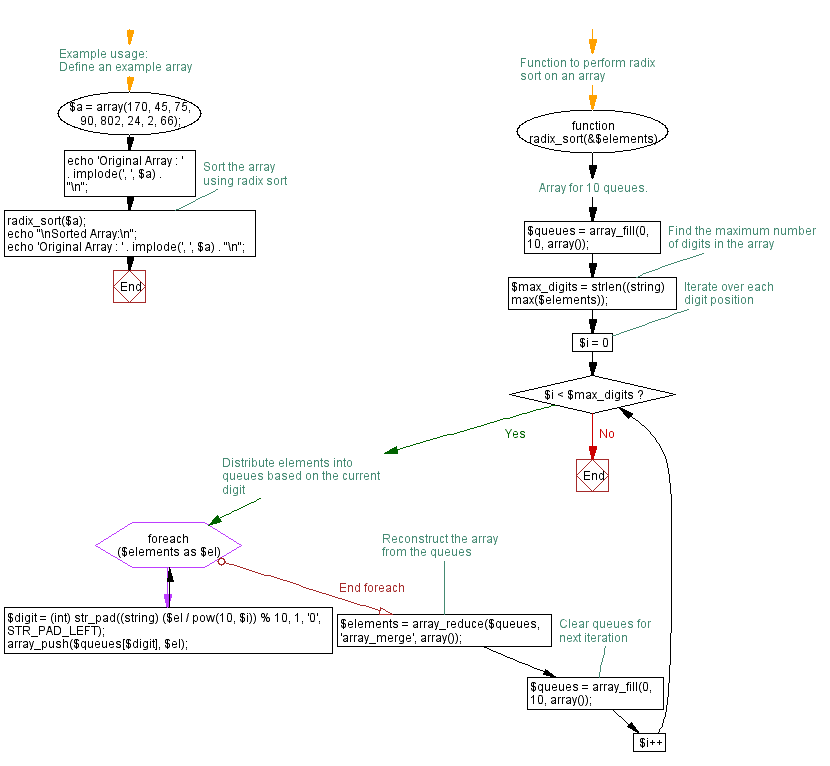﻿ PHP: Sort a list of elements using Radix sort - w3resource

# PHP Searching and Sorting Algorithm: Radix sort

## PHP Searching and Sorting Algorithm: Exercise-12 with Solution

Write a PHP program to sort a list of elements using Radix sort.

Sample Solution :

PHP Code :

``````<?php
// Array for 10 queues.
\$queues = array(
array(), array(), array(), array(), array(), array(), array(), array(),
array(), array()
);
// Queues are allocated dynamically. In first iteration longest digits
// element also determined.
\$longest = 0;
foreach (\$elements as \$el) {
if (\$el > \$longest) {
\$longest = \$el;
}
array_push(\$queues[\$el % 10], \$el);
}
// Queues are dequeued back into original elements.
\$i = 0;
foreach (\$queues as \$key => \$q) {
while (!empty(\$queues[\$key])) {
\$elements[\$i++] = array_shift(\$queues[\$key]);
}
}
// Remaining iterations are determined based on longest digits element.
\$it = strlen(\$longest) - 1;
\$d = 10;
while (\$it--) {
foreach (\$elements as \$el) {
array_push(\$queues[floor(\$el/\$d) % 10], \$el);
}
\$i = 0;
foreach (\$queues as \$key => \$q) {
while (!empty(\$queues[\$key])) {
\$elements[\$i++] = array_shift(\$queues[\$key]);
}
}
\$d *= 10;
}
}
// Example usage:
\$a = array(170, 45, 75, 90, 802, 24, 2, 66);
print_r(\$a);
print_r(\$a);
?>
```
```

Sample Output:

```Array
(
 => 170
 => 45
 => 75
 => 90
 => 802
 => 24
 => 2
 => 66
)
Array
(
 => 170
 => 45
 => 75
 => 90
 => 802
 => 24
 => 2
 => 66
)
```

Flowchart :PHP Code Editor:

Have another way to solve this solution? Contribute your code (and comments) through Disqus.

What is the difficulty level of this exercise?

Test your Programming skills with w3resource's quiz.

﻿